# Growing with Mathematics

Growing with Mathematics incorporates the best practices from the literature related to teaching young children and mathematics education.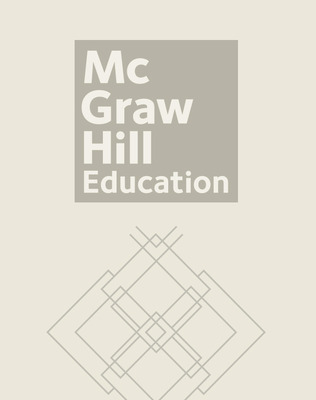Growing With Math's development was informed by the key research that has provided insights into how children learn and the vast amount of information known about the development of mathematical concepts and skills in the elementary grades.

## Program Components

•Growing With Math, 100 Number Line Grades 2-4
•Growing with Math, 200 Number Line Grade 3
•Growing with Math, Grade 2, Calendar and Data Kit
•Growing with Math, Grade 2, Comprehensive Classroom Kit (Includes Classroom Kit and Manipulative Kit)
•Growing with Math, Grade 2, Math Chat
•Growing with Math, Grade 3, Classroom Kit
•Growing with Math, Grade 3, Comprehensive Classroom Kit (Includes Classroom Kit and Manipulative Kit)
•Growing with Math, Grade 3, Discussion Book - Student
•Growing with Math, Grade 3, Resource Kit
•Growing with Math, Grade 4, Discussion Book - Student
•Growing with Math, Grade 5, Classroom Kit
•Growing with Math, Grade 5, Comprehensive Classroom Kit (Includes Classroom Kit and Manipulative Kit)
•Growing with Math, Grade 1, Student Book 1
•Growing with Math, Grade 1, Student Book 1
•Growing with Math, Grade 1, Student Book 2
•Growing with Math, Grade 2, Student Book 1
•Growing with Math, Grade 2, Student Book 1
•Growing with Math, Grade 2, Student Book 2
•Growing with Math, Grade 3, Student Book 1
•Growing with Math, Grade 3, Student Book 2
•Growing with Math, Grade 4, Student Book 1
•Growing with Math, Grade K, Student Book
•Growing with Math, Grade Pre-K, Literature: Milly and Molly Concept Lap Book
•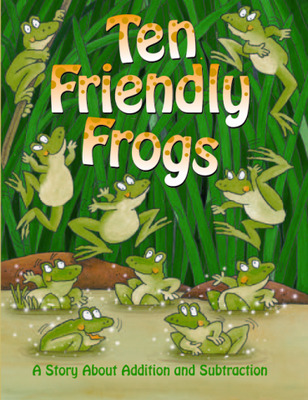Growing with Math, Grade 1, Math Literature: Ten Friendly Frogs Big Book (Addition & Subtraction)
•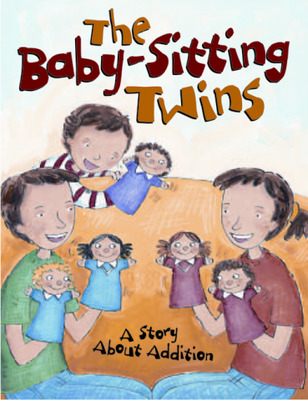Growing with Math, Grade 1, Math Literature: The Babysitting Twins Big Book (Addition)
•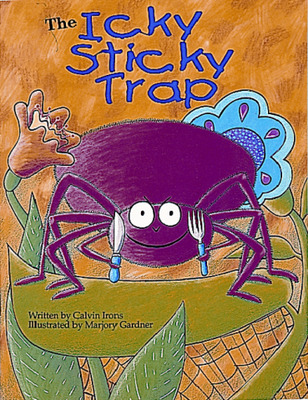Growing with Math, Grade 1, Math Literature: The Icky Sticky Trap Big Book (Subtraction)
•Growing with Math, Grade 1, Math Literature: The Pitterpats Big Book (Patterns)
•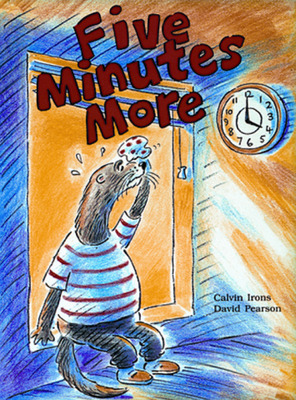Growing with Math, Grade 2, Math Literature: Five Minutes More Time Big Book (Time Past the Hour)
•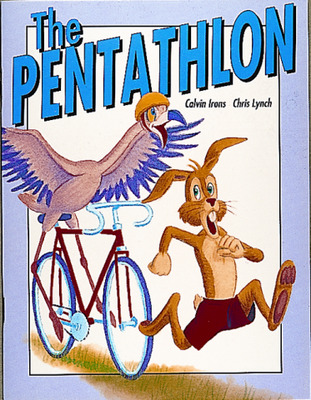Growing with Math, Grade 2, Math Literature: The Pentathlon Big Book (Addition & Subtraction)
•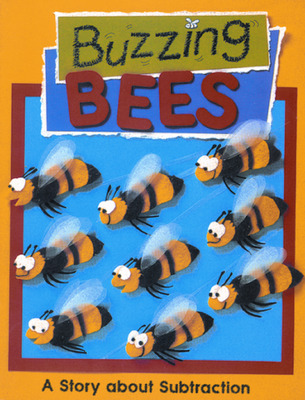Growing with Math, Grade K, Math Literature: Buzzing Bees Big Book (Subtraction)
•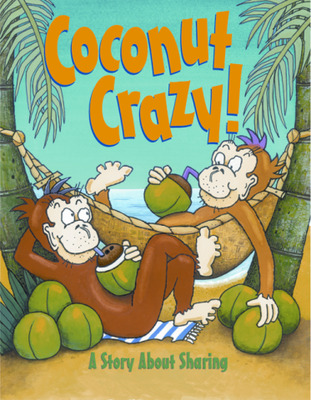Growing with Math, Grade K, Math Literature: Coconut Crazy! Big Book (Sharing)
•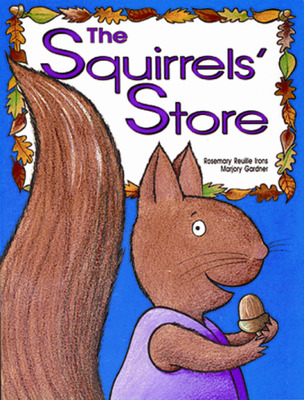Growing with Math, Grade K, Math Literature: The Squirrels' Store Big Book (Equal Groups)
•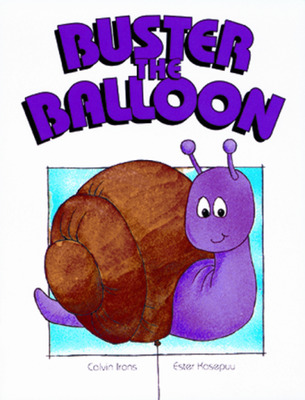Growing with Math, Grade Pre-K, Math Literature: Buster the Balloon Big Book (Comparing Sizes)
•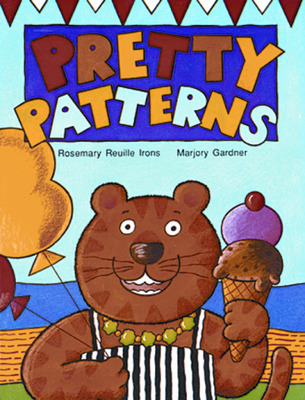Growing with Math, Grade Pre-K, Math Literature: Pretty Patterns (Patterning), Big Book
•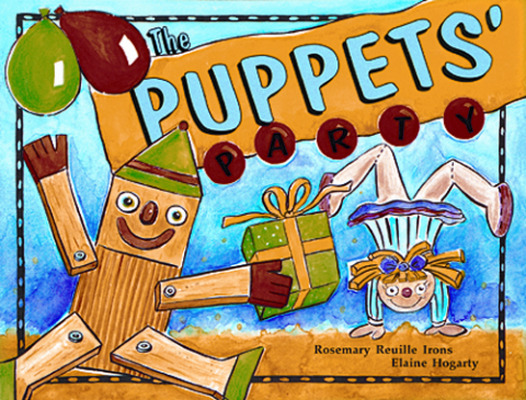Growing with Math, Grade Pre-K, Math Literature: The Puppets' Party (Counting to Ten), Big Book
Sort by: